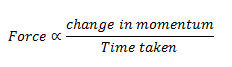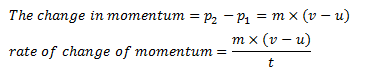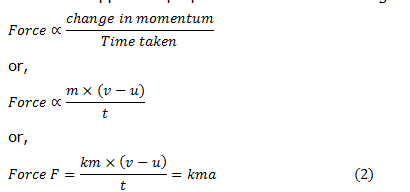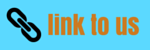# Laws Of Motion

## 6. Second Law of motion

• Newton's second law of motion gives the relationship between the force and acceleration.
• The second law of motion states that
the rate of change of momentum of an object is proportional to the applied unbalanced force in the direction of force
• The rate of change of momentum of an object is proportional to the applied force. So, Newton's second law of motion can be expressed as• Suppose an object of mass, $m$ is moving along a straight line with an initial velocity, $u$. It is uniformly accelerated to velocity, $v$ in time, $t$ by the application of a constant force, $F$ throughout the time, $t$.
• The initial and final momentum of the object will be, p1 = mu and p2 = mv respectively.
• Now change in momentum would be• Now force applied is proportional to rate of change of momentum. So,where, a =(v-u)/t is the acceleration which is the rate of change of velocity. The quantity,k is a constant of proportionality
• The unit of force is chosen in such a way that the value of the constant, k becomes one.
• For this, one unit of force is defined as the amount that produces an acceleration of 1 ms-2 in an object of 1 kg mass. That is,
1 unit of force = k × (1 kg) × (1 m s-2). Thus, the value of k becomes 1.
• From Eq. 2
F = ma           (3)
• The unit of force is kgms-2 or Newton, which has the symbol N.
• The second law of motion gives us a method to measure the force acting on an object as a product of its mass and acceleration.

## 7. Third Law of motion

• Newton's third law of motion states that
Whenever a body exerts a force on another body, the second body exerts an equal and opposite force on the first body
• So according to third law of motion to every action there is an equal and opposite reaction. This tells us that all forces in nature acts in pairs.
• These two forces are always equal in magnitude but opposite in direction.
• These forces act on different objects and never on the same object.
• Few applications of third law of motion are
1. If you throw a heavy ball or block away from your body, the force exerted will push back on you, possibly pushing you backward onto the ground.
2. A book lying on a table exerts a force on the table which is equal to the weight of the book (action force). The table supports the book, by exerting an equal force on the book (reaction). As the system is at rest, net force on it is zero. Therefore, forces of action and reaction must be equal and opposite.
3. When a gun is fired, the bullet moves forward (action). The gun recoils backwards (reaction).